• 【Numpy基础】np.interp()用法
2021-10-20 16:33:47

np.interp()主要使用场景为一维线性插值，个人理解的主要作用就是拟合曲线，根据现有的曲线取点来进行拟合。

## 参考文献

numpy.interp()用法python
更多相关内容
• 目录一、a.cumsum()二、np.interp()三、np.maximum.accumulate四、np.trapz 一、a.cumsum() a.cumsum(axis=None, dtype=None, out=None) 参数 a :数组 axis: 轴索引,整型，若a为n维数组，则axis的取值范围为[0,n-1] ...

# 一、a.cumsum()

a.cumsum(axis=None, dtype=None, out=None)

参数
a :数组
axis: 轴索引,整型，若a为n维数组，则axis的取值范围为[0,n-1]
dtype: 返回结果的数据类型，若不指定，则默认与a一致n
out: 数据类型为数组。用来放置结果的替代输出数组，它必须具有与输出结果具有相同的形状和缓冲长度

返回
沿着指定轴的元素累加和所组成的数组，其形状应与输入数组a一致

1、对于一维数组而言

import numpy as np

arr=np.array([1,2,3,4,5,6,7,8,9])
result=arr.cumsum()    #此时axis只能取0，因此，axis=0可不写

#result： array([ 1,  3,  6, 10, 15, 21, 28, 36, 45], dtype=int32)


2、对于二维数组而言

import numpy as np

arr=np.array([[1,2,3],[4,5,6],[7,8,9]])

#沿着axis=0轴计算

result1=arr.cumsum(0)   #array([[ 1,  2,  3],[ 5,  7,  9],[12, 15, 18]], dtype=int32)

#沿着axis=1轴计算

result2=arr.cumsum(1)   #array([[ 1,  3,  6],[ 4,  9, 15],[ 7, 15, 24]], dtype=int32)

#arr.cumsum()并不是arr.cumsum(0)和arr.cumsum(1)的并集，而是将arr重塑为一维数组后的，再计算cumsum()的结果

arr.cumsum()#array([ 1,  3,  6, 10, 15, 21, 28, 36, 45], dtype=int32)


# 二、np.interp()

np.interp()是一个一维线性插值函数。

np.interp(x, xp, fp, left=None, right=None, period=None)

参数
x: 数组 待插入数据的横坐标
xp: 一维浮点数序列 原始数据点的横坐标，如果period参数没有指定那么就必须是递增的 否则，在使用xp = xp % period正则化之后，xp在内部进行排序
fp: 维浮点数或复数序列 原始数据点的纵坐标，和xp序列等长.
left: 可选参数，类型为浮点数或复数（对应于fp值） 当x < xp时的插值返回值，默认为fp.
right: 可选参数，类型为浮点数或复数（对应于fp值），当x > xp[-1]时的插值返回值，默认为fp[-1].
period: None或者浮点数，可选参数 横坐标的周期 此参数使得可以正确插入angular x-coordinates. 如果该参数被设定，那么忽略left参数和right参数

返回
浮点数或复数（对应于fp值）或ndarray. 插入数据的纵坐标，和x形状相同

注意
在没有设置period参数时，默认要求xp参数是递增序列

实例1、插入一个值

import numpy as np
import matplotlib.pyplot as plt

x = 2.5
xp = [1, 2, 3]
fp = [3, 2, 0]
y = np.interp(x, xp, fp)  # 1.0
plt.plot(xp, fp, '-o')
plt.plot(x, y, 'x')
plt.show()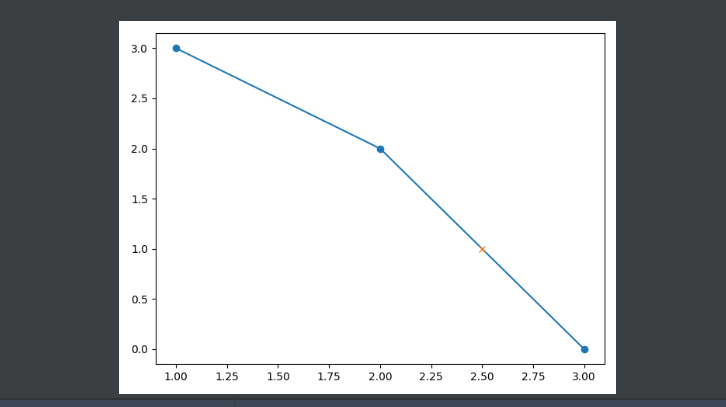实例二、插入一个序列

import numpy as np
import matplotlib.pyplot as plt

x = [0, 1, 1.5, 2.72, 3.14]
xp = [1, 2, 3]
fp = [3, 2, 0]
y = np.interp(x, xp, fp)  # array([ 3. ,  3. ,  2.5 ,  0.56,  0. ])
plt.plot(xp, fp, '-o')
plt.plot(x, y, 'x')
plt.show()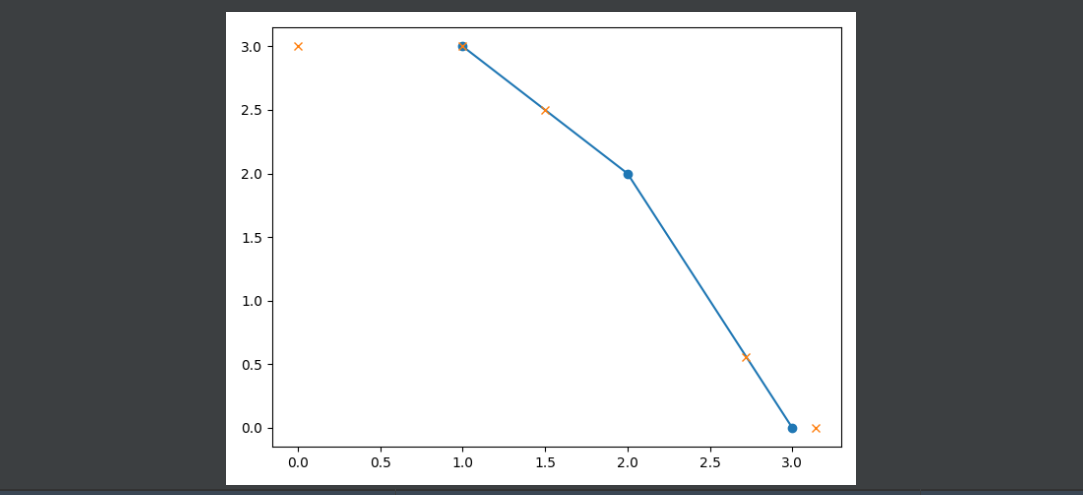# 三、np.maximum.accumulate

计算数组（或数组的特定轴）的累积最大值

例子

import numpy as np

d = np.array([2, 0, 3, -4, -2, 7, 9])
c = np.maximum.accumulate(d)
print(c)   # array([2, 2, 3, 3, 3, 7, 9])


# 四、np.trapz

numpy.trapz(y, x=None, dx=1.0, axis=-1)

计算y, x两者围成的面积（梯形）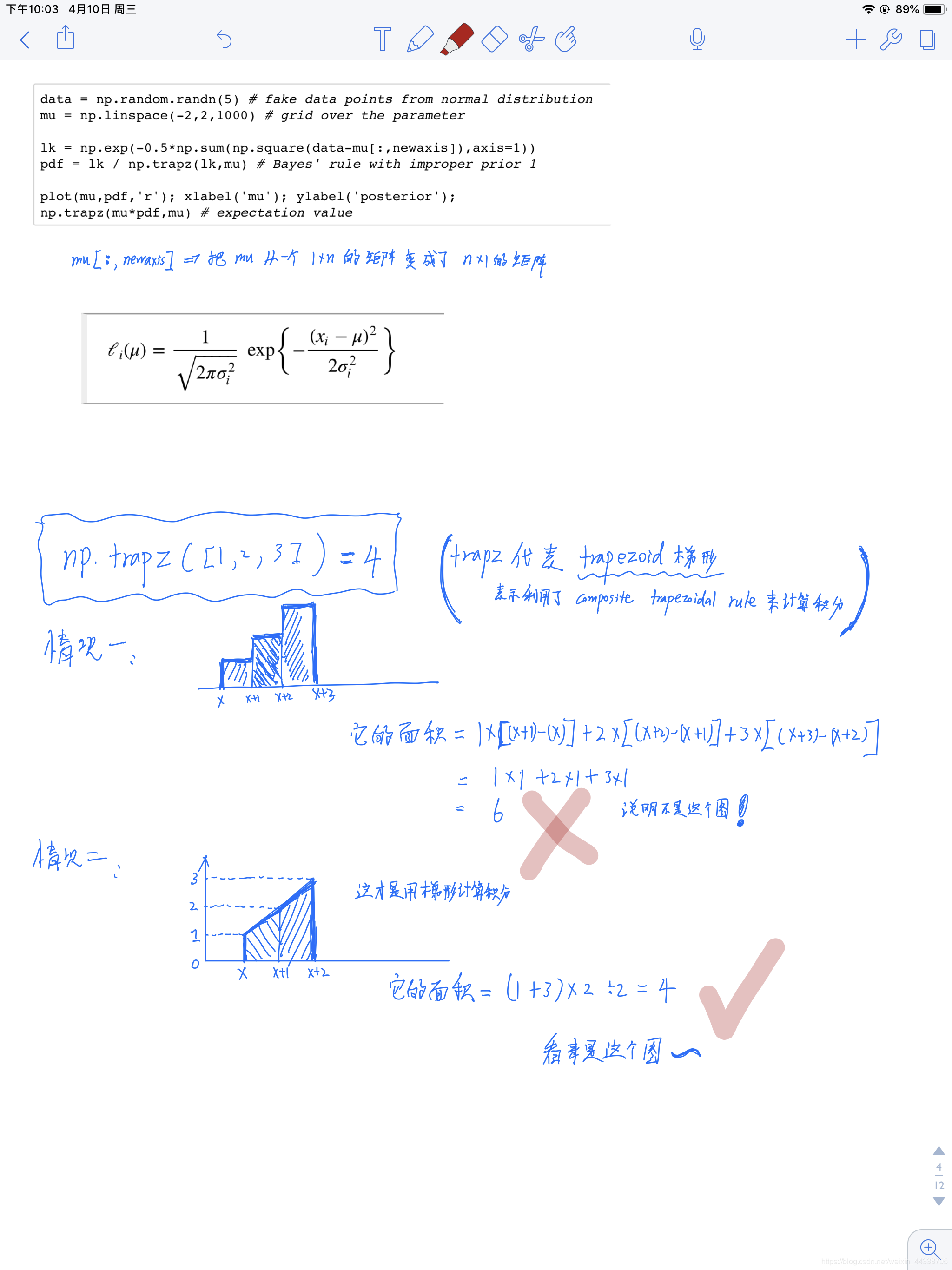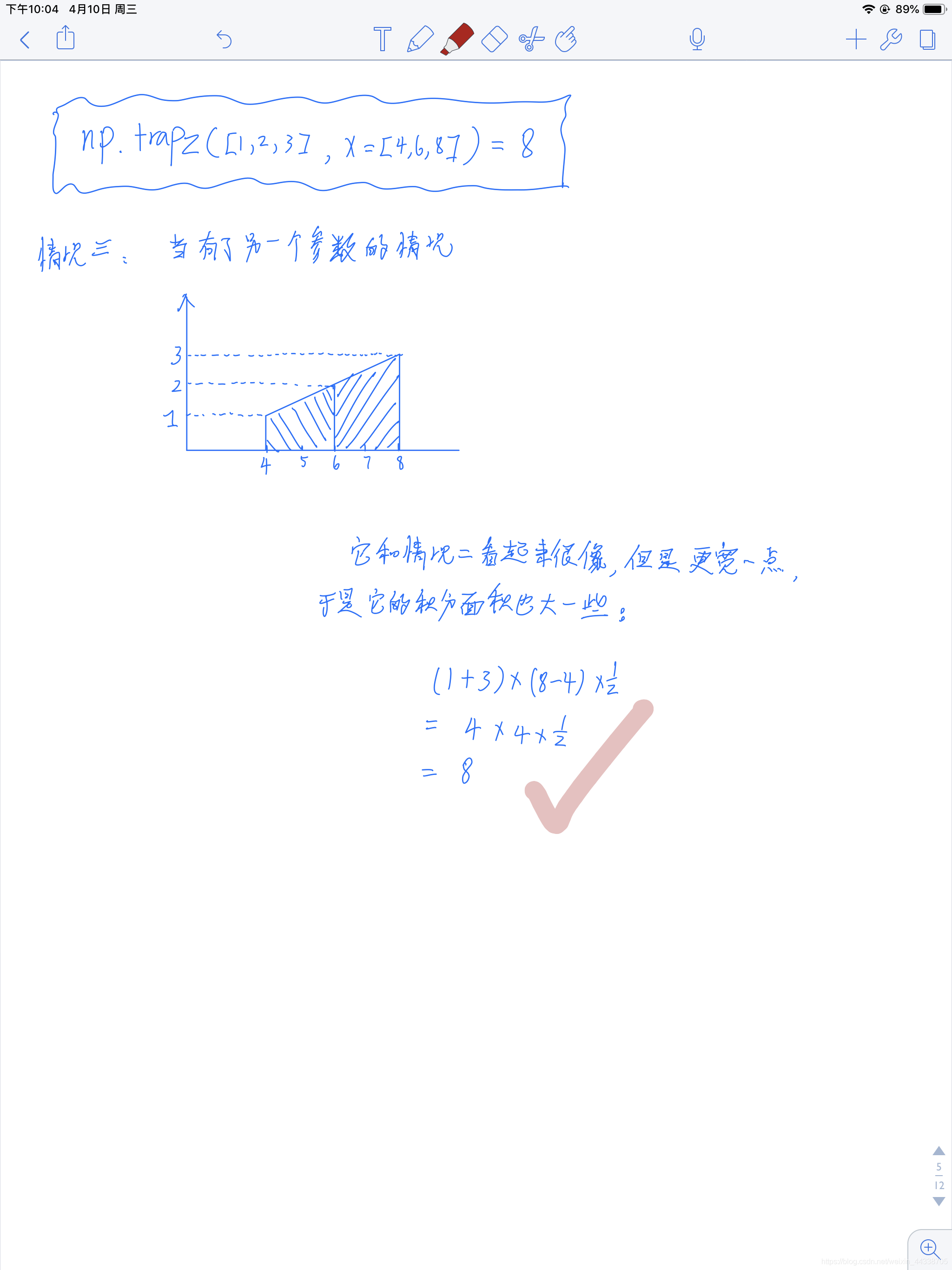展开全文python numpy
• 该方法是用于取值，建立XY轴的线性关系 具体参考 numpy.interp()用法_MrLittleDog的博客-CSDN博客_np.interp
展开全文numpy
• ## 线性插值 np.interp()

千次阅读 2020-05-26 05:40:35
线性插值是指插值函数为一次多项式的插值方式，其在插值节点上的插值...y = np.interp(x, xp, fp) x: 数组 待插入数据的横坐标. xp: 一维浮点数序列 原始数据点的横坐标，如果period参数没有指定那么就必须是递增的。否

线性插值是指插值函数为一次多项式的插值方式，其在插值节点上的插值误差为零。线性插值相比其他插值方式，如抛物线插值，具有简单、方便的特点。线性插值的几何意义即为概述图中利用过A点和B点的直线来近似表示原函数。

线性插值法是认为现象的变化发展是线性的、均匀的，所以可利用两点式的直线方程式进行线性插值。估算的是两点之间的点的对应值。

y = np.interp(x, xp, fp)
x: 数组 待插入数据的横坐标.

xp: 一维浮点数序列
原始数据点的横坐标，如果period参数没有指定那么就必须是递增的。否则，在使用xp = xp % period正则化之后，xp在内部进行排序.

fp: 一维浮点数或复数序列，原始数据点的纵坐标，和xp序列等长.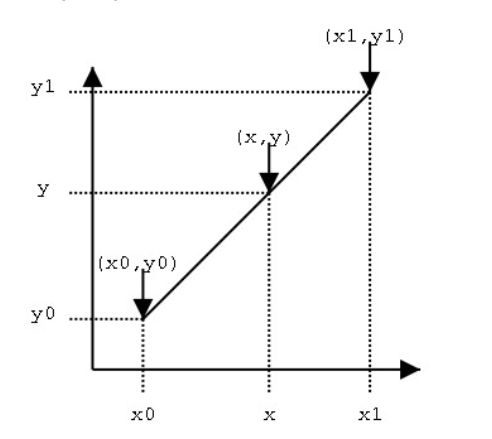即由(x0,y0)和(x1,y1)得到(x,y)。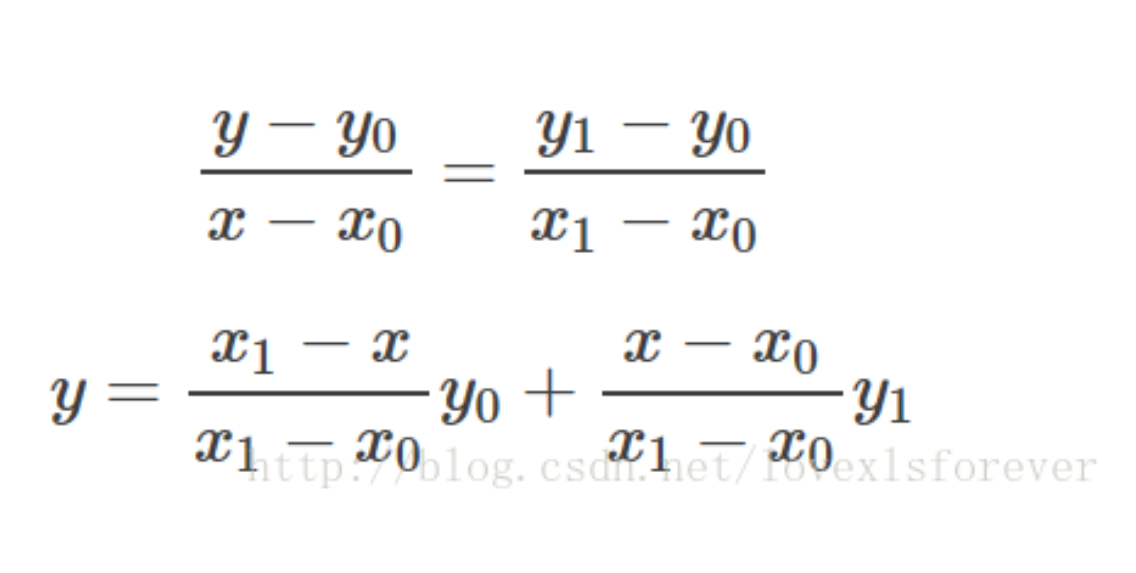## 双线性插值==也就是执行两次线性插值

双线性插值是有两个变量的插值函数的线性插值扩展，其核心思想是在两个方向分别进行一次线性插值
先通过Q12和Q22得到R2，Q21和Q11得到R1，然后由R1和R2得到P。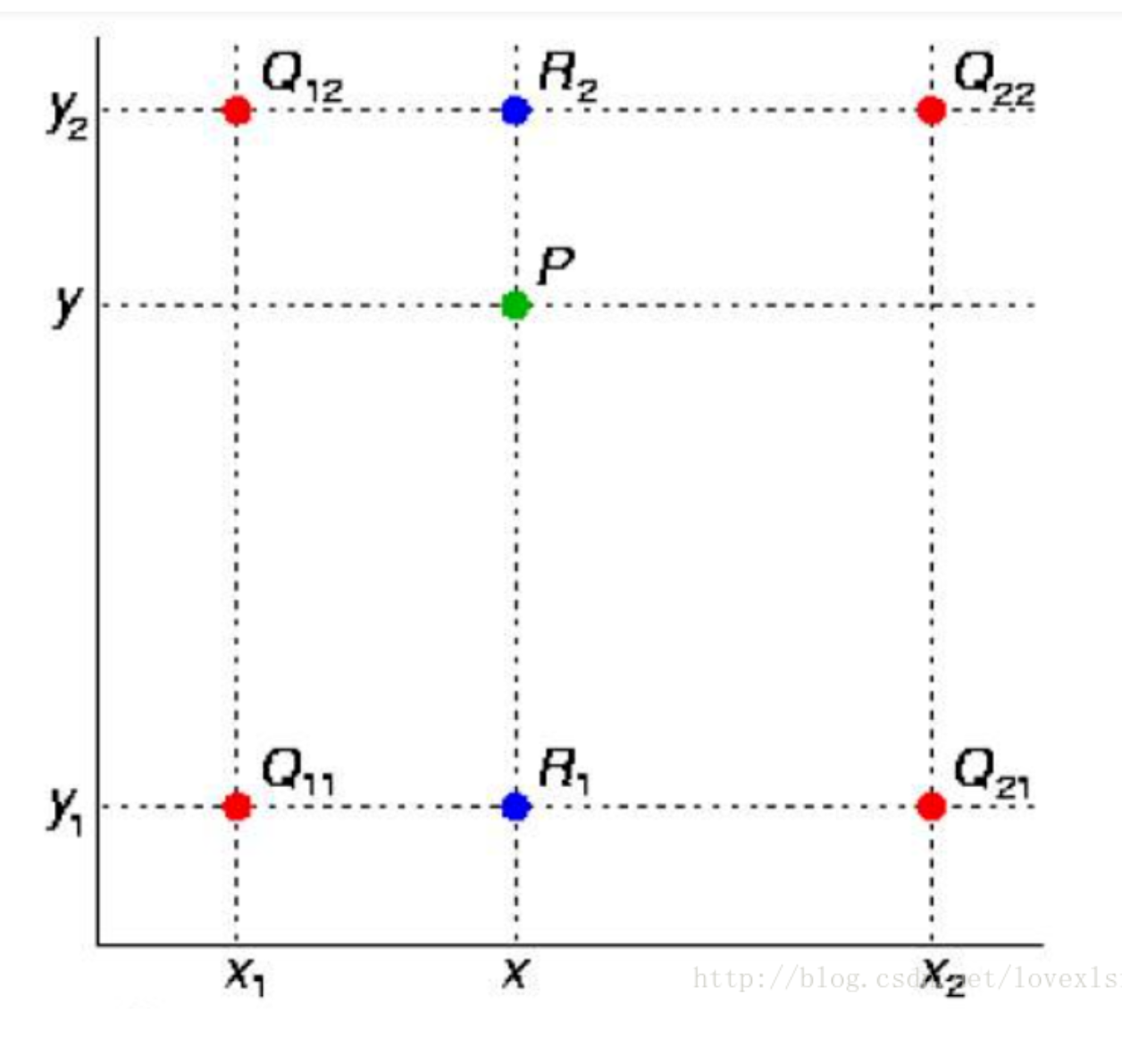假如我们想得到未知函数 f 在点 P = (x, y) 的值，假设我们已知函数 f 在 Q11 = (x1, y1)、Q12 = (x1, y2), Q21 = (x2, y1) 以及 Q22 = (x2, y2) 四个点的值。最常见的情况，f就是一个像素点的像素值。首先在 x 方向进行线性插值，得到

只要填入Q11的x以及Q12的x，就可以得到对应的纵坐标f。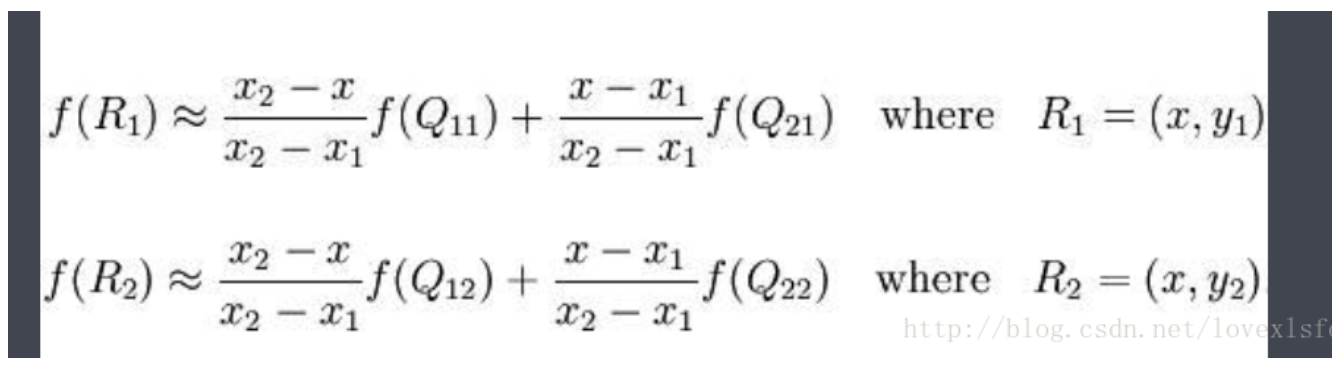只要填入P的纵坐标，就剋以得到P的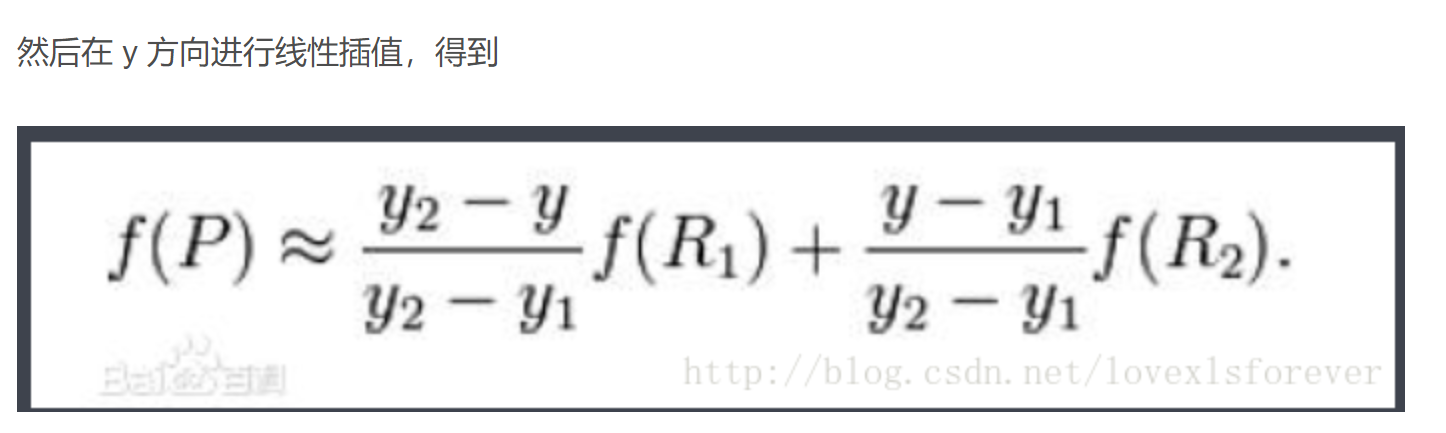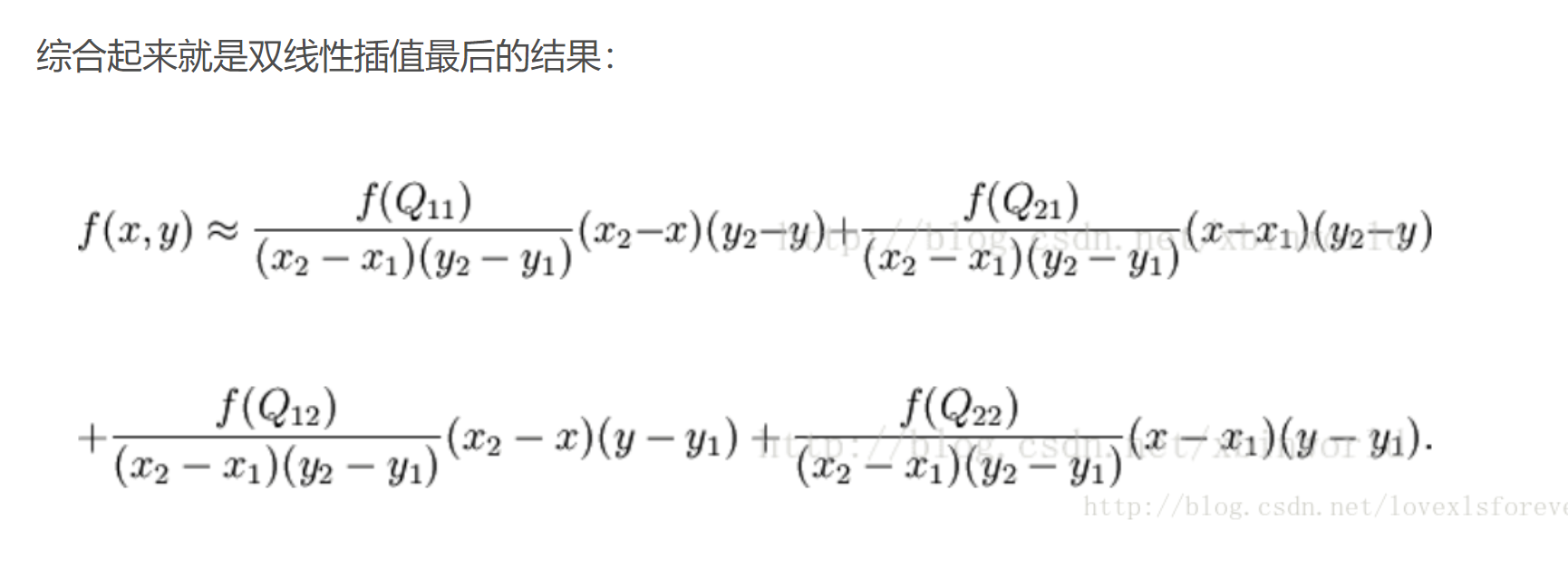展开全文• python——numpy——np.interp()python 机器学习
• 本文整理汇总了Python中numpy.interp方法的典型用法代码示例。如果您正苦于以下问题：Python numpy.interp方法的具体用法？Python numpy.interp怎么用？Python numpy.interp使用的例子？那么恭喜您, 这里精选的方法...
• ## numpy.interp()用法

万次阅读 多人点赞 2019-02-15 20:51:56
numpy.interp()主要使用场景为一维线性插值，我在直接看官方文档时一下子没有明白，后来结合图像绘制才明白它的用法，下面我们使用官方代码示例和我给出的图像对其进行简单介绍。 首先官方对该函数的解释是： 一维...python 线性插值
• ## 浅谈numpy.interp用法

千次阅读 2020-08-13 21:26:27
numpy.interp是线性插值，是一元函数插值，也就是说自变量只有一个。假设自变量是x，变量是y，同时已知x0,x1,...,xn,y0,y1,...,yn的值,且xi与yi是一一对应的关系，用Python的数据结构List来包裹，就是xp=[x0,x1,...,...numpy
• scipy.interpolate.interp2d注意事项python插值方法scipy.interpolate.interp2d注意事项问题scipy.interpolate.interp2d的使用变化说明 python插值方法scipy.interpolate.interp2d注意事项 问题 在使用scipy....python 人工智能
• scipy.interpolate.interp2d插值函数 博客地址：https://editor.csdn.net/md?not_checkout=1&articleId=109588264 1. interp2d函数用法 详见官方API文档 2. 问题描述 Python 3.8.3 Platform: Spyder 3 SciPy ...python scipy numpy
• 我试图得到一个三次样条函数scipy.interpolate.interp1d功能。我试图让documentation page上的示例正常工作，但每当我运行它时，都会出现以下错误：plt.plot(x,y,'o',xnew,f(xnew),'-', xnew, f2(xnew),'--') File"/...
• 所以看看我的猜测，我试...所以对于下一个有我同样问题的假人来说，这就是我想要的：In : interp_data = interpolate.interp1d(data[:,0], data[:,1:], axis=0, kind='cubic')In : data_int = np.zeros((len...
• 接上一篇脚本中有个错误，一直未解决，其实是interpolate.interp2d的输入参数错误，输入参数应该一维数组，而不是二位数组。参考...
• 例如,我有一个二维数据数组,其中一个维度上带有误差条,如下所示：In : numpy as npIn : x = np.linspace(0,10,5)In : y = np.sin(x)In : y_er = (np.random.random(len(x))-0.5)*0.1In : data = np....
• class scipy.interpolate.interp1d(x, y, kind='linear', axis=-1, copy=True, bounds_error=None, fill_value=nan, assume_sorted=False) 解释一 import numpy as np import matplotlib.pyplot as plt from scipy ...
• SciPy的interpolate模块提供了许多对数据进行插值运算的函数，范围涵盖简单的一维插值到复杂多维插值求解。当样本数据变化归因于一...classscipy.interpolate.interp1d(x,y,kind='linear',axis=-1,copy=True,bounds_...
• scipy.interpolate.interp1d (x, y, kind=‘linear’, axis=-1, copy=True, bounds_error=None, fill_value=nan, assume_sorted=False)` api中对于参数 y 有这么一句话: A N-D array of real values. The ...
• Numpy一维线性插值函数 ...numpy.interp(x, xp, fp, left=None, right=None, period=None) x - 表示将要计算的插值点x坐标 xp - 表示已有的xp数组 fp - 表示对应于已有的xp数组的值 left -...
• >最近“捕捉”到最近的数据点. >零是零阶样条.它的价值在任何时候都是最后看到的原始..., kinds): new_y = interpolate.interp1d(x, y, kind=kind)(new_x) ax.plot(new_x, new_y, 'ro-') ax.set_title(kind) plt.show()
• Python 插值通常我们在文献中看到很多色彩比较光滑的图，...一维、二维例子和代码如下：01.pyimport numpy as npfrom scipy import interpolateimport matplotlib.pyplot as pltx = np.linspace(0, 4, 12)y = np.cos...python插值
• def interp(x, xp, fp, left=None, right=None, period=None): Parameters ---------- x : array_like The x-coordinates at which to evaluate the interpolated values. xp : 1-D sequence of floats The x...python numpy 程序人生
• numpy.interp()主要使用场景为一维线性插值，返回离散数据的一维分段线性插值结果。 参数： x: 数组 待插入数据的横坐标. xp: 一维浮点数序列 原始数据点的横坐标，如果period参数没有指定那么就必须是递增的。...
• 根据 scipy.interpolate.interp2d 中对于interp2d的用法进行了介绍。 class scipy.interpolate.interp2d(x, y, z, kind='linear', copy=True, bounds_error=False, fill_value=nan) 函数参数 x, y : array_like ...计算机视觉 算法 机器学习
• matlab中interp1对应scipy.interpolate y=interp1(F(:,1),F(:,2),ES_band_double') 样本点存放在F数组中，待插值的数据为ES_band_double，样本数据shape为496*2，ES_band_double的shape为1*137，...y = np.interp....